• 多元回归-最小二乘法-残差分析笔记 一.多元线性回归模型的假设 我们需要进行以下六个假设，这些假设是经典的多元线性回归模型有效的前提： 1、因变量Y和自变量X1，X2，…，Xk之间的关系是线性的。 2、自变量（X1，X2...
• 主要介绍了scikit-learn线性回归，多元回归，多项式回归的实现，文中通过示例代码介绍的非常详细，对大家的学习或者工作具有一定的参考学习价值，需要的朋友们下面随着小编来一起学习学习吧
• 多元回归分析(Multiple Regression Analysis)是指在相关变量中将一个变量视为因变量，其他一个或多个变量视为自变量，建立多个变量之间线性或非线性数学模型数量关系式并利用样本数据进行分析的统计分析方法。...
• 关于一元回归分析和多元回归分析的matlab代码，含有测试数据，直接就可以运行，然后出图，注释清楚，通俗易懂，每一步都有注释，比较详细。
• 2 2 Matlab 实现多元回归实例 一一般多元回归 一般在生产实践和科学研究中人们得到了参数 x ?x1,xn ? 和因变量 y 的数据需要求出关系式 y ? f ? x ? 这时就可以用到回归分析的方法如果 只考虑 f 是线性函数的情形当...
• https://blog.csdn.net/weixin_39739342/article/details/93379653 完整的数据文件及py文件
• 文章应用股市中三个具有典型意义的技术指标,RSI,KDJ和5日平均线建立了非线性回归预测模型,对股票的价格走势进行了短期预测。所建立的回归模型对预测某些股票的短期价格趋势提供了参考,具有一定的理论价值和实际应用...
• 相关向量机做回归预测分类都能实现，回归预测使用RVR类，分类使用RVC。 RVM是支持向量机的稀疏贝叶斯模拟，具有许多优点： 它提供了概率估计，而不是SVM的点估计。 通常提供比SVM更稀疏的解决方案，后者倾向于使支持...
• Prepared by the multiple regression of cross-validation procedure
• 多元门限回归模型优于单纯多元回归模型，曹琨，，利用乌江渡1951-2006年月径流量和74项气象因子资料拟合，建立单纯的多元回归模型和非线性多元门限回归模型，对2007、2008年月径流量进�
• ## 多元回归

千次阅读 2019-04-13 21:02:58
多元回归 多元线性回归模型的基本假定 为了方便地进行模型的参数估计，我们对以下回归方程式有如下假定： Y^=Xβ+ε\hat{Y}=X\beta+\varepsilonY^=Xβ+ε 解释变量x1,x2,⋯&ThinSpace;,xpx_1,x_2,\cdots,x...


文章目录
多元回归多元线性回归模型的基本假定参数估计量的性质回归方程的显著性检验中心化、标准化和相关系数与偏相关系数小结

多元回归
多元线性回归模型的基本假定
为了方便地进行模型的参数估计，我们对以下回归方程式有如下假定：

Y

^

=

X

β

+

ε

\hat{Y}=X\beta+\varepsilon

解释变量

x

1

,

x

2

,

⋯
&ThinSpace;

,

x

p

x_1,x_2,\cdots,x_p

是确定性变量，不是随机变量，且要求

r

a

n

k

(

X

)

=

p

+

1

&lt;

n

rank(X)=p+1&lt;n

。这里的

r

a

n

k

(

X

)

=

p

+

1

&lt;

n

rank(X)=p+1&lt;n

，表明设计矩阵

X

X

中的自变量列之间不相关，样本量的个数应该大于解释变量的个数，

X

X

是以满秩矩阵。随机误差项具有零均值等方差，即

{

E

(

ε

i

)

=

0

，

i

=

1

,

2

,

⋯
&ThinSpace;

,

n

c

o

v

(

ε

i

,

ε

j

)

=

{

σ

2

,

i

=

j

0

,

i

≠

j

,

i

,

j

=

1

,

2

,

⋯
&ThinSpace;

,

n

\begin{cases} E(\varepsilon_i)=0，\quad i =1,2,\cdots,n\\ cov(\varepsilon_i,\varepsilon_j)= \begin{cases} \sigma^2, &amp;{i = j} \\ 0, &amp; {i\neq j} \end{cases}\quad,i,j=1,2,\cdots,n \end{cases}

这个假定常称为Gauss-Markov条件。

E

(

ε

i

)

=

0

E(\varepsilon_i)=0

，即假设观察值没有系统误差，随机误差项

ε

i

\varepsilon_i

的平均值为零。随机误差项

ε

i

\varepsilon_i

的协方差为零，表明误差项在不同点之间是不相关的（在正态假定下即为独立的），不存在序列相关，并且有相同的精度。
参数估计量的性质
多元线性回归方程未知

β

0

,

β

1

,

.

.

.

,

β

p

\beta_0,\beta_1, ..., \beta_p

的估计是用最小二乘估计得到的。

y

^

=

X

β

^

=

X

(

X

′

X

)

−

1

X

′

y

\hat{y}=X\hat{\beta}=X(X^{&#x27;}X)^{-1}X^{&#x27;}y

中的

H

=

X

(

X

′

X

)

−

1

X

′

H=X(X^{&#x27;}X)^{-1}X^{&#x27;}

是一个帽子矩阵,可以证明其是

n

n

阶对称阵，同时还是幂等矩阵，即

H

=

H

2

H=H^2

。以下说一下参数估计量的性质：

β

^

\hat{\beta}

是随机向量

y

y

的线性变换。

β

^

\hat{\beta}

是

β

\beta

的无偏估计。

D

(

β

^

)

=

σ

2

(

X

′

X

)

−

1

D(\hat{\beta})=\sigma^2(X^{&#x27;}X)^{-1}

高斯-马尔可夫定理：在假定的

E

(

y

)

=

X

β

,

D

(

y

)

=

σ

2

I

n

E(y)=X\beta,D(y)=\sigma^2I_n

时，

β

\beta

的任一线性函数

c

′

β

c^{&#x27;}\beta

的最小方差线性无偏估计

(

B

L

U

E

)

(BLUE)

为

c

′

β

c^{&#x27;}\beta

，其中，

c

c

是任一

p

+

1

p+1

维常数向量，

β

^

\hat{\beta}

是

β

{\beta}

的最小二乘估计。 读者在阅读高斯-马尔可夫定理的时候应该注意一下几点：可能存在非线性的，有偏估计使得方差不是最小。在正态假定下，即不可能存在

y

1

,

y

2

,

⋯
&ThinSpace;

,

y

n

y_1,y_2,\cdots,y_n

非线性函数,，也不可能存在

y

1

,

y

2

,

⋯
&ThinSpace;

,

y

n

y_1,y_2,\cdots,y_n

的其他非线性函数，作为

c

′

β

c^{&#x27;}\beta

的无偏估计，比最小二乘估计

c

′

β

^

c^{&#x27;}\hat{\beta}

的方差更小。

c

o

v

(

β

^

,

e

)

cov(\hat{\beta},e)

=0当

y

=

N

(

X

β

,

σ

2

I

n

)

y = N(X\beta,\sigma^2I_n)

时，则

β

^

\hat{\beta}

~

N

(

β

,

σ

2

(

X

′

X

)

−

1

)

N(\beta,\sigma^2(X^{&#x27;}X)^{-1})

S

S

E

σ

2

\frac{SSE}{\sigma^2}

~

X

2

(

n

−

p

−

1

)

X^2(n-p-1)

回归方程的显著性检验
最小二乘法只是定性地给出了线性回归模型，但模型是否有效或者回归系数是否显著还需要经过检验才行。一般对线性回归模型进行两项统计检验：1、回归方程显著性

F

F

检验。2、回归系数显著性

t

t

检验。同时介绍衡量回归拟合程度的拟合优度检验。

F

F

检验

F

F

检验的原假设是:

H

0

:

β

1

=

β

2

,

⋯
&ThinSpace;

,

β

p

=

0

H_0 :\beta_1=\beta_2,\cdots,\beta_p=0

,当

F

&gt;

F

α

(

p

,

n

−

p

−

1

)

F&gt;F_{\alpha}(p,n-p-1)

时，拒接原假设，认为在显著水平

α

\alpha

下,

y

y

与

x

1

,

x

2

,

⋯
&ThinSpace;

,

x

p

x_1,x_2,\cdots,x_p

有显著关系，即方程回归显著的。也可以看它的

p

p

值，当

p

&lt;

α

p&lt;\alpha

时,拒绝原假设。

t

t

检验

t

t

检验的目是检验单个回归系数是否显著的，从而达到剔除多余无效变量的作用。注意由于各个变量之间是有交互作用的，因此不能一次剔除多个不显著变量，原则上每次只剔除一个变量，先剔除其中

t

t

值最小的（或者

p

p

值最大的）一个变量，然后再对求的得的新的回归回归方程进行检验，有不显著的变量再剔除，直到保留的变量对

y

y

有显著影响为止。在一元回归中，这两个检验是等价的，但是在多元检验中两者是不等价的。  拟合优度 拟合优度用于检验回归方程对样本观察值的拟合程度。样本决定系数：

R

2

=

S

S

R

S

S

T

=

1

−

S

S

E

S

S

T

R^2=\frac{SSR}{SST}=1-\frac{SSE}{SST}

当样本量

n

n

和回归变量数目差接近的时候，

R

2

R^2

接近1，隐含虚假成分，因此在用这个决定系数决定模型的优劣一定要慎重。
中心化、标准化和相关系数与偏相关系数
在解释中心化之前先介绍一下舍入误差，及病态矩阵的概念。舍入误差主要是四舍五入等导致的误差，产生舍入误差产生的主要原因有二：①数据之间的量级悬殊；②设计矩阵

X

X

的列向量近似线性相关，导致

X

′

X

X^{&#x27;}X

为病态矩阵，其逆矩阵

(

X

′

X

)

−

1

(X^{&#x27;}X)^{-1}

就会产生较大的误差。病态矩阵：方程

A

X

=

B

AX=B

当其解集对

A

A

高度敏感，那么称

A

A

为病态矩阵。为了解决数据量纲和舍入误差的影响，因此要中心化和标准化。
1.中心化 中心化变换只改变直线截距，不改变直线斜率。通过坐标系的平移变换，使坐标原点移至样本中心，即做变换：

{

x

j

′

=

x

i

j

−

x

j

ˉ

y

i

′

=

y

i

−

y

ˉ

\begin{cases}{x_j}^{&#x27;}=x_{ij}-\bar{x_j}\\{y_i}^{&#x27;}=y_i-\bar{y}\end{cases}

2.标准化及标准化回归系数 为了消除量纲不同和数量级的差异带来的影响，就需要将数据标准化处理。让后利用得到标准化处理后的数据进行最小二乘估计位置参数，得到标准化回归系数。 3.复相关系数

R

R

反映的是

y

y

与一组自变量之间的相关关系，是整体和共性指标简单相关系数反映两个自变量间的相关性，是局部和个性指标。 4.偏相关系数可以度量

p

+

1

p+1

个变量

y

,

x

1

,

x

2

,

⋯
&ThinSpace;

,

x

p

y,x_1,x_2,\cdots,x_p

之中任意两个变量的线性程度，而这种线性相关程度实在固定其余

p

+

1

p+1

个变量的影响下的线性相关。
小结
我们一般用最小二乘法进行模型的参数估计，不过在之前，我们要检验样本数据是否满足高斯-马尔可夫条件。初步得到模型以后，我们要做的是检验模型是否反映了线性关系，模型是否有效，模型参数是否显著。模型不通过可能有以下几方面的原因：①问题应该用非线性取描述，而错误地用线性进行拟合；由于认识的局限性，把重要的自变量给露了。用

R

2

R^2

进行的时候，要注意自变量个数要远小于样本量个数，这时的拟合优度检验才不会出现虚假因素。在存在交互现象的时候，用控制变量的方法解释单个参数对模型的影响是不合理的。
展开全文• 根据某矿影响煤层瓦斯含量的大量参数值,运用多元回归分析的数学模型,建立了煤层瓦斯含量预测的多元回归方程,并对方程及其系数的显著性作了检验,进行了学生化残差分析。通过此数学模型能够直观地反映煤层埋深、煤层...
• 测试的多元回归数据 学校第 22 卷心理学认知能力测试 1985 年 1 月 使用 WISC-R 和 WOODCOCK-JOHNSON 认知能力测试的多重回归数据 LEADELLE PHELPS MARTIN ROSS0 FALASCO University of Missouri-Missouri Division ...
• 基于聚类分析和多元回归的空气质量的分析的R代码，包含聚类分析代码，因子分析代码，气泡图，柱形图。文章内容发布在我的博客中，希望对大家有所帮助
• 基于灰色多元回归耦合模型的武汉市“十三五”住房需求预测，吴良国，杨勃文，为了合理预测武汉市十三五期间住房需求量，本文应用灰色关联分析方法，分析了武汉市居民住房需求与各影响因素的关系，确定了影响
• 主要介绍了详解基于Jupyter notebooks采用sklearn库实现多元回归方程编程，文中通过示例代码介绍的非常详细，对大家的学习或者工作具有一定的参考学习价值，需要的朋友们下面随着小编来一起学习学习吧
• 考虑了多元回归的回归收缩和选择问题。 目标是始终如一地识别与回归相关的变量。 这不仅适用于预测变量，也适用于响应。 为此，发现了多元回归和典型相关之间的新关系。 随后，构造了其等效最小二乘类型公式，然后...
• 适合初学者学习理解多元回归分析模型的例子，通俗易懂
• matlab多元回归与曲线拟合编程学习。喜欢的下载
• 基于多元回归和Tiebout模型的云服务定价优化方法.pdf
• 用C#写的多元回归算法，有详细的注释，一看就明白。Java可以直接拿去更改，包含了一份data.xlsx文件数据。
• title: 数学建模—多元回归分析 tags: 建模 EverydayOneCat 木星全貌???? 知识点 1.笔记 在了接受域中，接受X为0的假设，X对外没有比较显著的线性关系。 2.知识点补充 多元回归模型：含两个以上解释变量的回归...
EverydayOneCat
木星全貌🪐知识点
1.笔记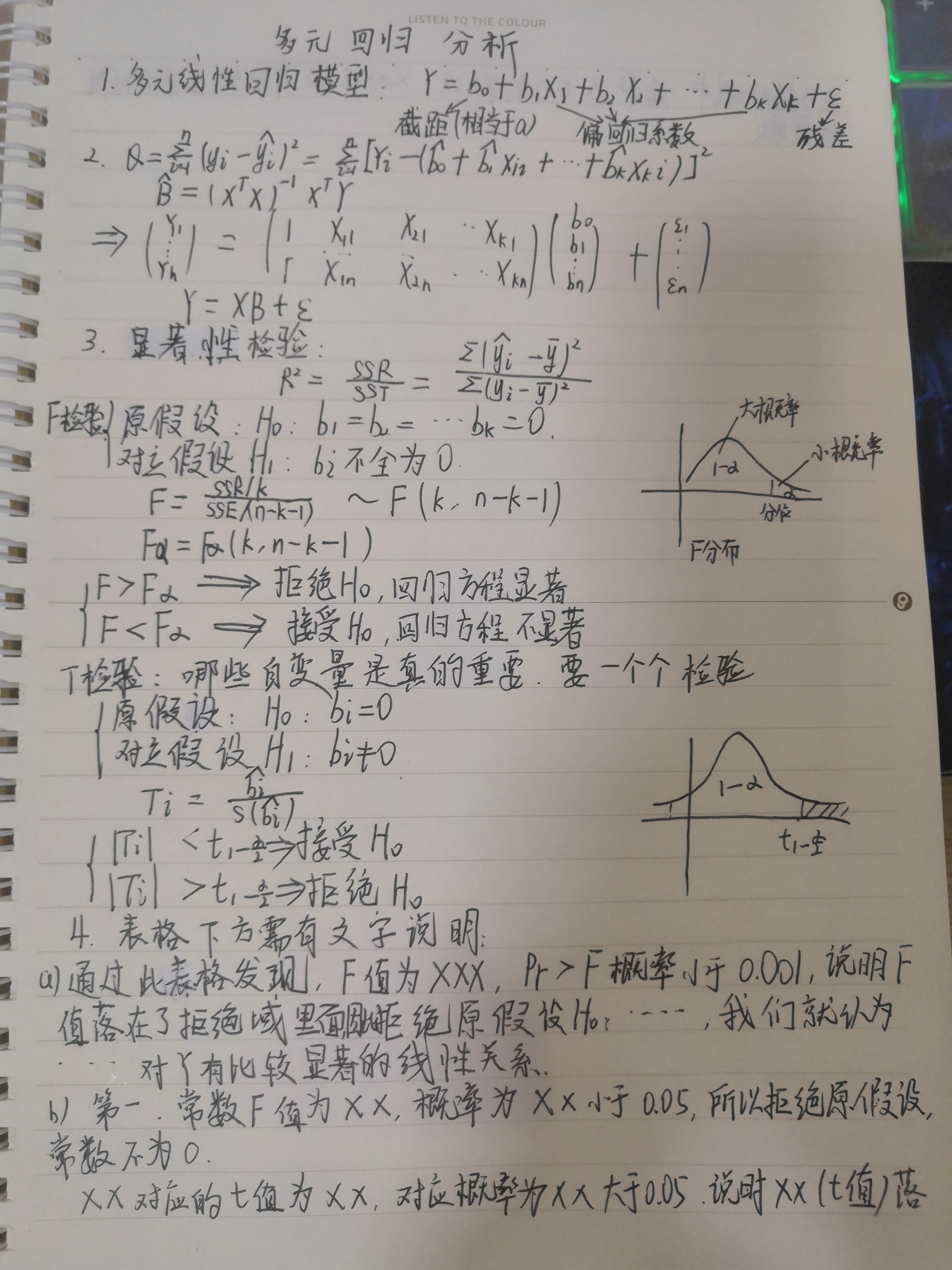在了接受域中，接受X为0的假设，X对外没有比较显著的线性关系。
2.知识点补充
多元回归模型：含两个以上解释变量的回归模型
多元线性回归模型的假设:
解释变量Xi 是确定性变量，不是随机变量解释变量之间互不相关，即无多重共线性随机误差项不存在序列相关关系随机误差项与解释变量之间不相关随机误差项服从0均值、同方差的正态分布
例题
1.多元线性回归
某品种水稻糙米含镉量y(mg/kg)与地上部生物量x1(10g/盆)及土壤含镉量x2(100mg/kg)的8组观测值如表。试建立多元线性回归模型。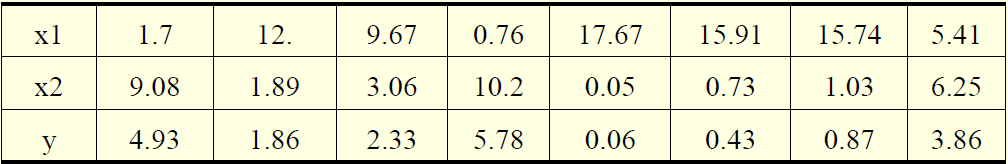1.1SAS代码
data ex;
input x1-x2 y@@;
cards;
1.7 9.08 4.93 12 1.89 1.86
9.67 3.06 2.33 0.76 10.2 5.78
17.67 0.05 0.06 15.91 0.73 0.43
15.74 1.03 0.87 5.41 6.25 3.86
;
proc reg;
model y=x1 x2;
run;

1.2结果分析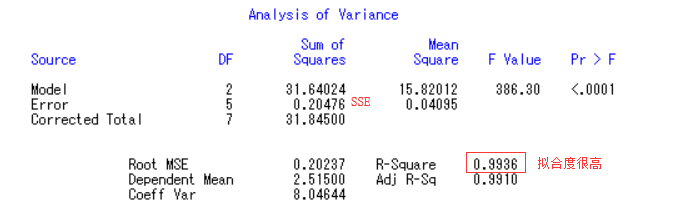我们可以通过WORD将这些复制做成表格更加美观直接，每个表下面都需要有文字说明：
由方差分析表可知，其F value=386.30,pr>F的值<0.0001，远小于0.05，说明F值落在了拒绝域里面，故拒绝原假设，接受备择假设，认为y1与x1,x2之间具有显著性的线性关系；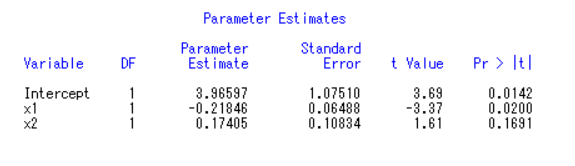由参数估计表可知，x2对应的t值为1.61，Pr>|t|的值=0.1691，大于0.05，说明1.61落在了接受域中，接受x2为0的假设，x2对外没有比较显著的线性贡献。
为此，需要在程序中model y1=x1 x2中去掉x2，再次运行：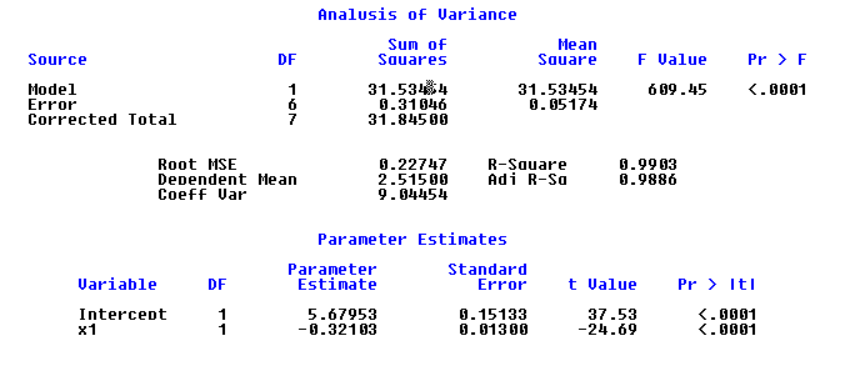对常数检验t值分别为t=37.53、,Pr>|t|的值<0.0001,远小于0.05，说明截距项通过检验，估计值为5.67953。 同理可知x1的系数通过检验，估计值为-0.32103
回归方程：y=-0.32103x1+5.67953
许多实际问题中可能还会出现某几个变量的系数并没有通过检验，此时，可以在原程序中的modely1=x1-x2中去掉没用通过的变量,直到所有的系数均通过检验。或者使用逐步回归方法，让软件自动保留通过检验的变量。
2.多元非线性回归

将非线性回归方程转化为线性回归方程。转化时应首先选择适合的非线性回归形式，并将其线性化。再确定线性化回归方程的系 数，最后确定非线性回归方程中未知的系数或参数。

湖北省油菜投入与产出的统计分析
1.投入指标 （1）土地（S）。土地用播种面积来表示。农作物播种面积是指当年从事农业 （2）劳动（L）。劳动用劳动用工数（成年劳动力一人劳动一天为一个工）来表示。劳动用工中包含着直接和间接生产用工。 （3）资本（K）。资本用物质费用来表示。物质费用包含直接费用和间接费用。主要有种子秧苗费、农家肥费、化肥费、农药费、畜力、固定资产折旧费和管理及其他费用等。
2.产出指标 产出指标用湖北省历年油菜生产的总产量(Y)来表示。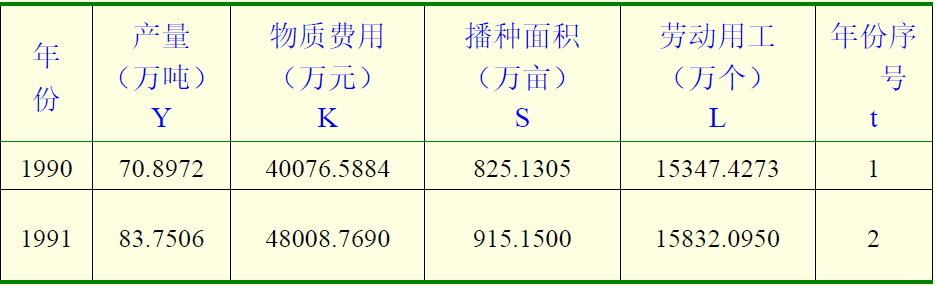2.1SAS代码
data ex;input y k s l t @@;
x1=log(k);x2=log(s);x3=log(l);y1=log(y);
cards;
70.8972 40076.5884 825.1305 15347.4273 1
83.7506 48008.7690 915.1500 15832.0950 2
70.8627 44593.8425 804.150 13306.8090 3
78.3451 43460.3229 783.2100 13314.5700 4
98.0749 72657.2633 923.8050 14596.1190 5
134.8767 146108.3421 1282.8900 20911.1070 7
147.5315 162433.3500 1244.7000 18670.5000 8
154.7607 166979.6325 1330.5150 18627.2100 9
159.9743 190395.5262 1505.4600 20775.3480 10
198.4942 205914.6645 1738.4100 22599.3300 11
194.7943 189762.7335 1677.0900 20963.6250 12
187.1013 193463.610 1761.9450 21936.2153 14
235.1184 183768.4035 1779.1500 19606.2330 15
;
proc reg;model y1=x1 x2 x3 t ; /*selection=stepwise*/
run;

2.2结果分析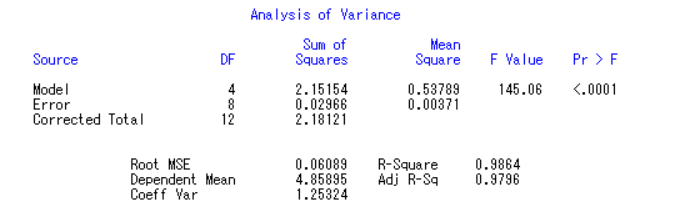F值为145.06，对应的Pr>F的概率小于0.001，说明F值落在了拒绝域中。故拒绝原假设H0:x1,x2,x3x,t都为0，x1,x2,x3x,t对y1有显著的线性关系。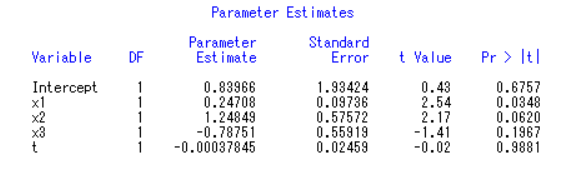这里我们遵循一个原则，先看变量，再看常数。变量如果要去，需要一个一个去，因为他们之间可能有线性关系，一个变量会影响另一个变量。
我们可以通过增加一段SAS代码查看变量之间的线性关系。
proc corr;var x1 x2 x3 t;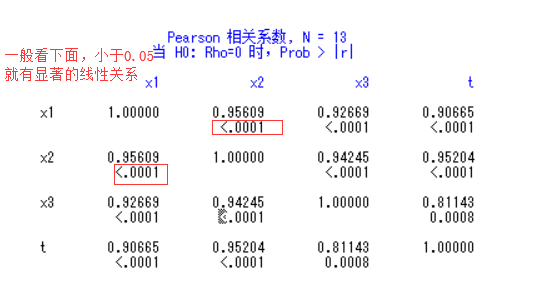可以看到，x1 x2 x3 t之间都有线性关系，这种其实是极不稳定的。
回到上面的表，我们看到常数显著性概率大于0.05，但是我们得先看变量，先不去管他。接着看x3,t都大于0.05很多，我们取最大的t显著性概率为0.9466，远大于0.05。因此将model y1=x1 x2 x3 t;去掉他，即改为model y1=x1 x2 x3 ;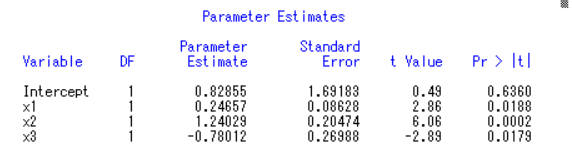截距项Intercept(常数)的显著性概率为0.6117，大于0.05，因此将model y1=x1 x2 x3 ; 改为model y1=x1 x2 x3/noint;（去掉常数项）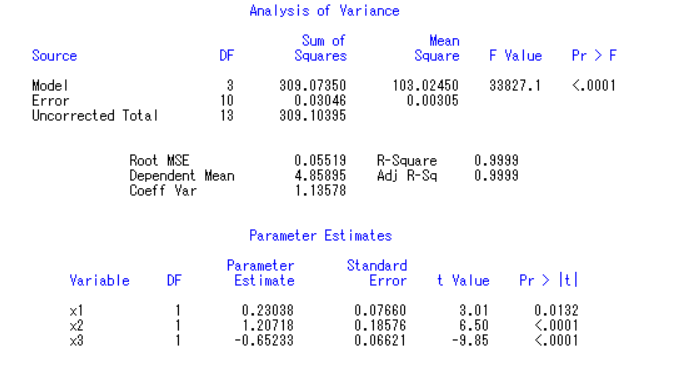这时候F检验也过了，T检验也过了。我们可以得出式子：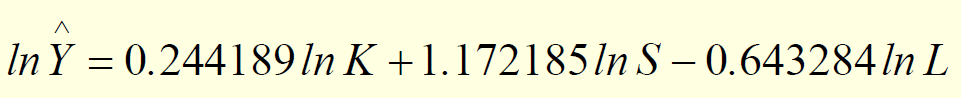F=34565.8 R2=0.9999 K，S，L的t值分别为（3.01） (6.59) (-9.98)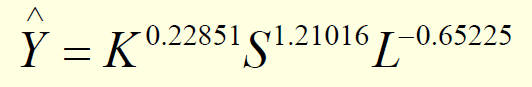但是我们通过经济学解释就会发现这个式子很不合理：K(资本)增长1%Y增长0.22851%，S(土地)增长1%Y增长1.21%，L(劳动)增长1%Y增长-0.65225%，而且不会随着时间t增长。弹性大于1，这个式子说明湖北省油菜产量主要靠土地来增长，显然不符合现实。本模型虽然满足数学规则，但不能通过经济检验。
上述说明我们刚刚对变量的逐一减法并不适用这一问题，我们可以依据经济学来修改模型：随着现代发展，劳动力其实对产量并无太大影响，反而年数增长带来的技术进步影响更为显著。
model y1=x1 x2 t/noint;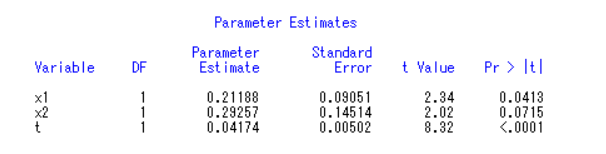虽然x2依然大于0.05，但是相差并不大，勉强可以算，这样才更符合现实。
3.逐步回归
逐步回归和上面对变量逐一减法想法，他是一个一个把变量加进去，不符合就删掉某个变量。
逐步回归选择变量快捷，但对于存在多重共线的自变量选择，有时并不准确，使用时注意分辨。
SAS使用逐步回归的方法是，模型从y1=x1开始，符合就加成y1=x1 x2，不符合就变成y1=x2…还有一种更为简单的方式，将model y1=x1 x2 x3 t ; 改为model y1=x1 x2 x3 t /selection=stepwise;机器自动判断。

注意，为了筛选变量宽容，程序中默认显著度为0.15，而不是0.05，以避免条件过于严格只用筛选无法进行。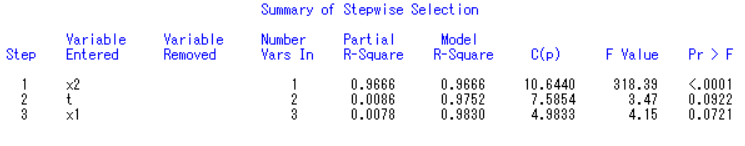从程序结果中不难看出，x2、x1、t进入模型。因此modely1=x1 x2 x3 t /selection=stepwise;改为model y1=x1 x2 t /noint;再运行一遍即可。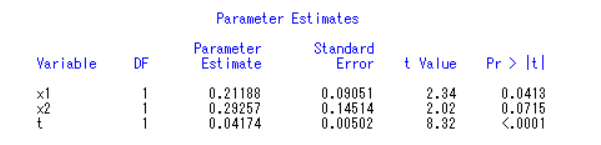4.标准化回归
由于单位量纲不一样，偏回归系数的大小不能完全反映自变量对因变量影响的大小。要想真实反映自变量的贡献，标准化回归是个好的选择。
标准化回归系数（Beta值）在多元回归中被用来比较变量间的重要性。
还是用上面的例子，将model y1=x1 x2 t /noint;改为model y1=x1 x2 t /noint stb;运行即可。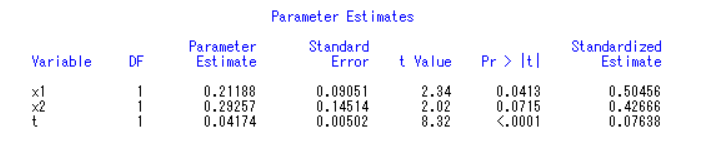从最后一列（标准化回归系数）可看出，x1重要性超过x2和t。与原先的参数大小比有变化。
同时这个也符合现实，在现实生活中，湖北省油菜很大的产量来自于资本的投资。
作业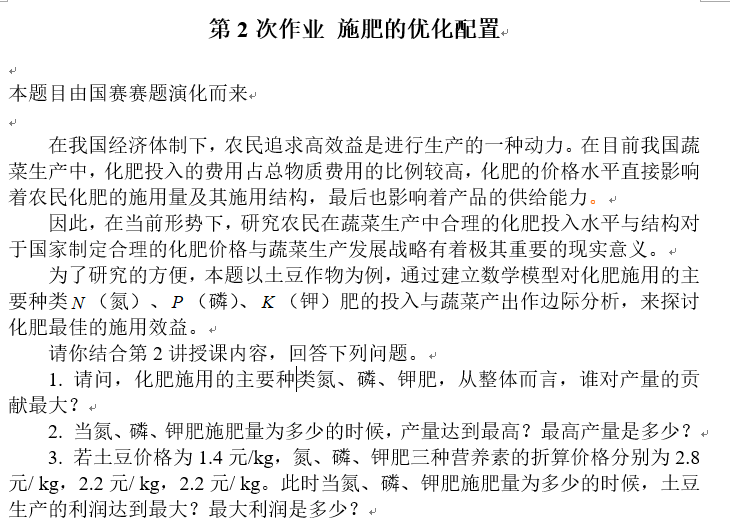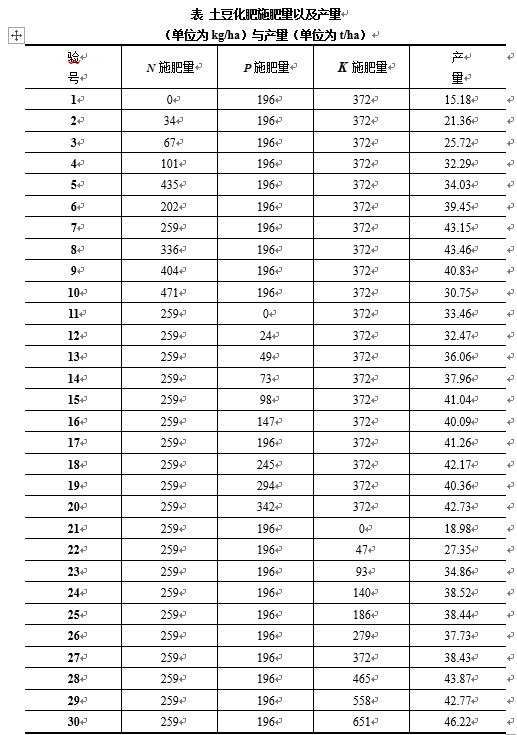1.问题一的分析与求解
为探究各种化肥（主要为N 、P 、K 肥）的投入量对于土豆产量的影响程度大小，我们决定使用多元回归模型，通过比较回归方程系数的大小来比较各种化肥的投入量对土豆产量影响程度的大小。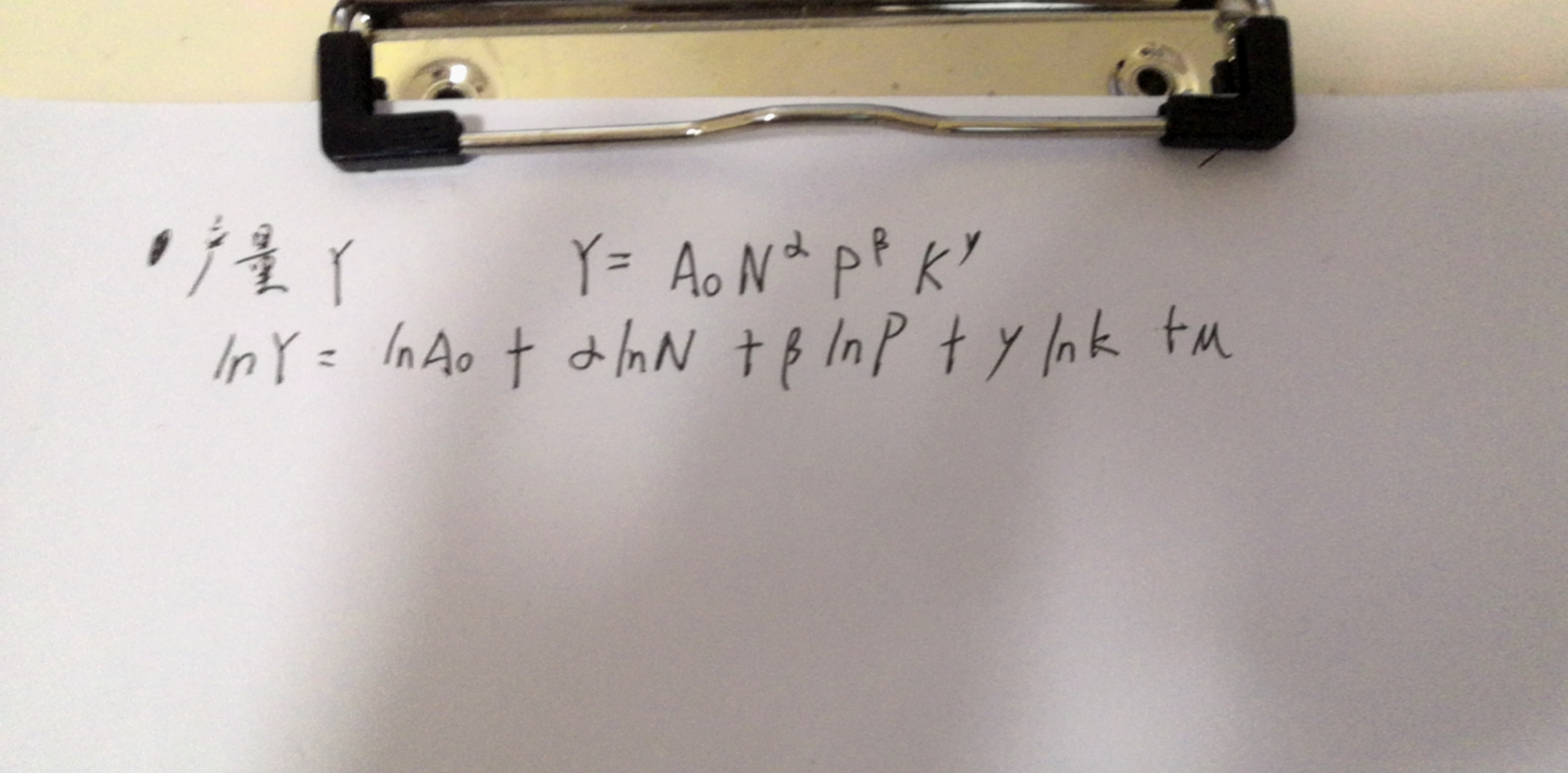编写SAS代码：
data ex;input n p k y@@;
x1=log(n);x2=log(p);x3=log(k);y1=log(y);
cards;
0 196 372 15.18
34 196 372 21.36
67 196 372 25.72
101 196 372 32.29
435 196 372 34.03
202 196 372 39.45
259 196 372 43.15
336 196 372 43.46
404 196 372 40.83
471 196 372 30.75
259 0 372 33.46
259 24 372 32.47
259 49 372 36.06
259 73 372 37.96
259 98 372 41.04
259 147 372 40.09
259 196 372 41.26
259 245 372 42.17
259 294 372 40.36
259 342 372 42.73
259 196 0 18.98
259 196 47 27.35
259 196 93 34.86
259 196 140 38.52
259 196 186 38.44
259 196 279 37.73
259 196 372 38.43
259 196 465 43.87
259 196 558 42.77
259 196 651 46.22
;
proc reg;model y1=x1 x2 x3;
proc corr;var x1 x2 x3;
run;

首先我们对自变量x1、x2、x3 之间的相关性进行判断，计算得相关系数如下表：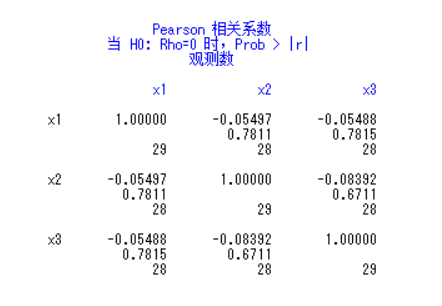通过相关系数表我们可以看出各自变量之间得相关系数的绝对值均小于0.09，因此可以认为自变量之间无相关性，使用多元回归分析效果较好。
接着使用最小二乘法对自变量和因变量进行多元回归分析，对得到的回归方程进行F检验，得到方差分析表：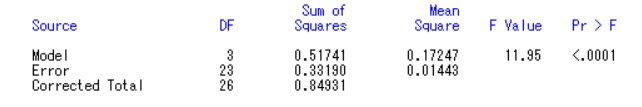由方差分析表可知，其 F value=11.95，对应的Pr>F的概率小于0.001，故11.95落在了拒绝域中，拒绝原假设H0：x1,x2,x3都为0。认为y1 与x1、x2、x3 之间有显著性的线性关系。
接着对各参数进行显著性检验，通过t 检验得到如下参数估计表：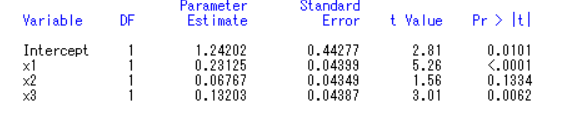由参数估计表可知：
（1）对自变量x1检验的t值为5.26，Pr>|t|的值小于0.001，因此拒绝原假设认为x1系数为0，说明x1的系数通过检验，x1与y1有显著的线性关系。
（2）对自变量x2 检验的t 值为t=1.56，Pr>|t|的值=0.1334，大于0.05，因此接受原假设认为x2 系数为0，说明x2 的系数没有通过检验，x2 与y 没有显著的线性关系，且其回归系数最小，即x2 对y 的影响不明显，因此在回归方程中去除自变量x2。
（3）对自变量x3 检验的t 值为t=3.01，Pr>|t|的值=0.0062，小于0.05，因此拒绝原假设认为x3 系数为0，说明x3 的系数通过检验，x1 与y 有显著的线性关系。
去除变量x2 后，继续对y 与x1、x3 进行回归分析，步骤同上。在方程通过F 检验后得到如下参数估计表：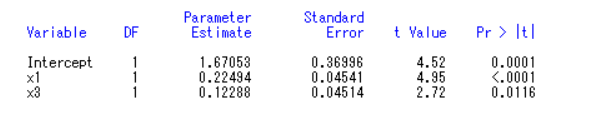但是我们考虑到现实生活，磷肥不可能毫无影响，这里我们注意，下面的题目是让我们预测，当我们做预测这种题型时，其实T检验没有那么严格，我们只需要保证F检验拒绝原假设就可以了。我们可以保留x2，增加一句话：x2对结果没有线性影响。
x1>x3>x2，综上所述，化肥种类对土豆产量影响程度由大到小的排列顺序为氮肥、钾肥和磷肥。
2.问题二的分析与求解

经验之谈：一般这种求因变量极值的问题建模都用二次函数

编写SAS代码：
data ex;input n p k y@@;
x1=n*n;x2=p*p;x3=k*k;x4=n*p;x5=n*k;x6=p*k;
cards;
/*同上数据区*/
;
proc reg;model y=n p k x1-x6;
run;

运行结果：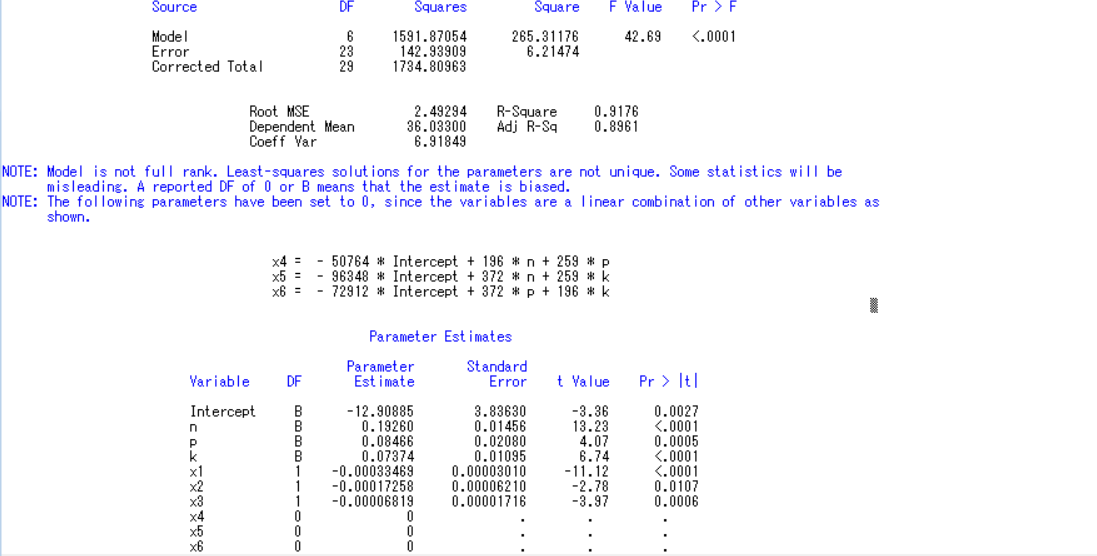我们发现运行后SAS警告我们x4,x5,x6和我们的n,p,k有线性关系，高度共线，也就是说，我们通过n,p,k完全可以表示x4,x5,x6：

x

4

=

−

50764

∗

I

n

t

e

r

c

e

p

t

+

196

∗

n

+

259

∗

p

x4 = - 50764 * Intercept + 196 * n + 259 * p

x

5

=

−

96348

∗

I

n

t

e

r

c

e

p

t

+

372

∗

n

+

259

∗

k

x5 = - 96348 * Intercept + 372 * n + 259 * k

x

6

=

−

72912

∗

I

n

t

e

r

c

e

p

t

+

372

∗

p

+

196

∗

k

x6 = - 72912 * Intercept + 372 * p + 196 * k

因此，需要去掉x4,x5,x6：model y=n p k x1-x3;再次运行，结果如下：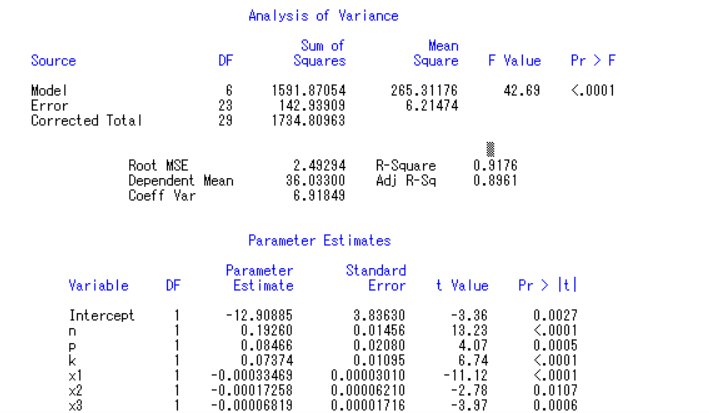我们发现无论是F检验还是T检验都很完美，符合度也高达91.76%，我们可以根据此写出我们的式子：

y

=

−

12.91

+

0.1926

∗

n

+

0.0847

∗

p

+

0.074

∗

k

−

0.00033469

∗

x

1

−

0.00017258

∗

x

2

−

0.00006819

∗

x

3

y=-12.91+0.1926*n+0.0847*p+0.074*k-0.00033469*x1-0.00017258*x2-0.00006819*x3

统计学检验通过了，我们需要来检验模型拟合精度，看误差率是多少。
SAS代码：
data ex;input n p k y@@;
x1=n*n;x2=p*p;x3=k*k;x4=n*p;x5=n*k;x6=p*k;
y1=-12.91+0.1926*n+0.0847*p+0.074*k-0.00033469*x1-0.00017258*x2-0.00006819*x3;
red=y-y1;wucha=abs(y-y1)/y*100;wc+wucha;
cards;
/*同上数据区*/
;
proc print;var y y1 red wucha wc;
run;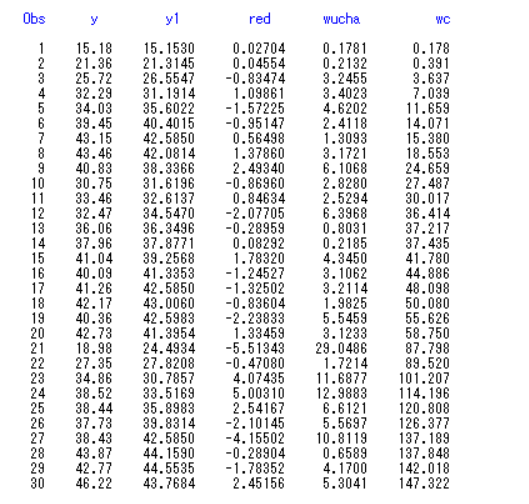我们发现平均误差率是147.322/30=4.9%左右，说明我们求得这个式子还算可以。接下来我们要Y最大，只需要对这三个变量求偏导即可求出，这里我们可以用LinGO软件。
max=y;
y=-12.91+0.1926*n+0.0847*p+0.074*k-0.00033469*n*n-0.00017258*p*p-0.00006819*k*k;
n*p=- 50764 * Intercept + 196 * n + 259 * p;
n*k=- 96348 * Intercept + 372 * n + 259 * k;
p*k=- 72912 * Intercept + 372 * p + 196 * k;

执行结果：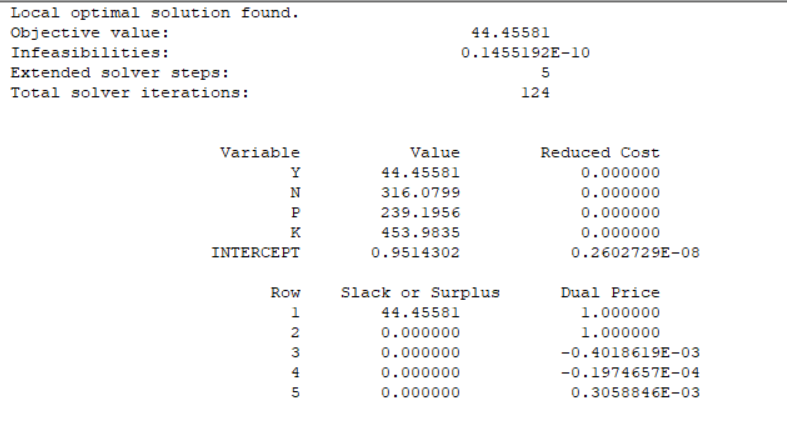可以看出，当氮肥316.0799kg，磷肥239.1956kg，钾肥453.9835kg时产量最高。
3.问题三的分析与求解
max=1.4*1000*y-2.8*n-2.2*p-2.2*k;
y=-12.91+0.1926*n+0.0847*p+0.074*k-0.00033469*n*n-0.00017258*p*p-0.00006819*k*k;
n*p=- 50764 * Intercept + 196 * n + 259 * p;
n*k=- 96348 * Intercept + 372 * n + 259 * k;
p*k=- 72912 * Intercept + 372 * p + 196 * k;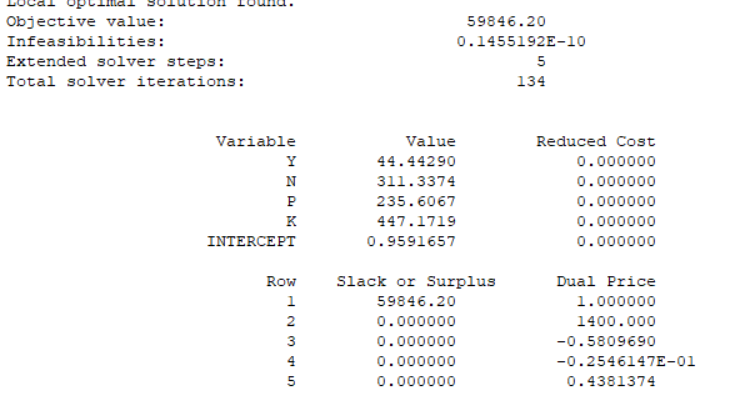结语高考的xdm加油！分享一首我高中高考冲刺的歌(●ˇ∀ˇ●)
展开全文数学建模
• 一元回归分析和多元线性回归 前言 在统计学中，回归分析(Regression Analysis)指的是确定两种或两种以上变量间的相互依赖的定量关系的一种分析方法。该方法常使用数据统计的基本原理，对大量统计数据进行数学处理...
一元回归分析和多元线性回归
前言
在统计学中，回归分析(Regression Analysis)指的是确定两种或两种以上变量间的相互依赖的定量关系的一种分析方法。该方法常使用数据统计的基本原理，对大量统计数据进行数学处理，并确定因变量与某些自变量的相关关系，建立一个相关性较好的回归方程（函数表达式），并加以外推，用于预测以后的因变量的变化的分析方法。回归分析是一种预测性的建模技术，它研究的是因变量（目标）和自变量（预测器）之间的关系。实际上预测分析是回归分析的一种。之前有说过分类问题，模式分类是通过构造一个分类函数或者分类模型将数据集映射到一个个给定的类别中（这些类别是离散的），它是模式识别的核心研究内容，关系到其识别的整体效率，广泛应用于各个研究领域。而回归问题则是确定两种或者两种以上变量间相互依赖的定量关系的一种统计方法。（这些结果是连续的）。就结果形式上来看，分类试图得到一个向离散集合的映射，回归试图得到一个向连续集合的映射。一个简单的回归方程：
用户满意度= 0.008*形象 + 0.645*质量 + 0.221*价格这个方程说明质量对用户满意度贡献比最大，质量提高1分，满意度提升0.645分，以此类推，形象贡献最小。使用回归分析得到这个式子，在今后产品上市前，可以根据产品的质量、价格以及形象预测用户对即将上市产品满意度。（这三个特征任意可以计算一个确切的值，即映射到一个连续不断的空间。） 回归分析方法较多，常见如下。
按照涉及变量多少，分为一元回归分析和多元回归分析。按照因变量的多少，分为简单回归分析和多重回归分析。按照自变量和因变量之间的关系类型，分为线性回归分析和非线性回归分析。  简介
一元回归分析
对于一组自变量x和对应的一组因变量y的值，x和y呈线性相关关系，现在需要求出满足这个线性关系的直线方程。在数学上一般使用最小二乘法，其主要思想就是找到这样一条直线，使所有已知点到这条直线的距离的和最短，那么理论上这条直线就应该和实际数据拟合度最高。 多元线性回归
上面的一元回归自变量只有一个，在回归分析中如果出现两个以上的自变量，称为多元回归。事实上，一种现象常常与多种因素关联，由多个自变量的最优组合共同来预测或估计因变量，比只用一个自变量进行预测或估计更有效，更符合实际。因此，多元线性回归意义大于一元回归。例如，一个消费水平的关系式中，工资水平、受教育水平、职业、地区、家庭负担等因素都会影响消费水平。  原理
一元回归分析
假设方程为

y

=

a

+

b

x

y=a+bx

已知很多数据点(x,y)，经过最小二乘法，计算出截距项a和斜率b。回归的目的就是建立一个回归方程来预测目标值，回归的求解就是求这个回归方程的回归系数，如a和b。在回归系数计算出来之后，预测的方法就非常简单了，只需要把输入值代入回归方程即可得到预测值。 多元线性回归
若特征X不止一个，可以构造多元线性回归模型。

y

=

a

0

+

a

1

x

1

+

a

2

x

2

+

.

.

.

y=a_0 + a_1x_1 + a_2x_2 + ...

多元线性回归与一元线性回归类似，可以用最小二乘法估计模型参数，也需要对模型及模型参数进行统计检验，计算出a0,a1,a2等参数。这时就可以得到多元回归方程，此时，给出多个自变量，使用回归方程，就可以预测因变量。 回归计算法
步骤
通过对Loss函数求每个参数的偏导。对所有偏导数，令其为0，解出参数值。 这种方法就是最小二乘法。对于多元回归，可以使用矩阵进行相关推导，大致思想与一元回归类似，使用最小二乘法。  实战
身高与体重的一元回归
运行结果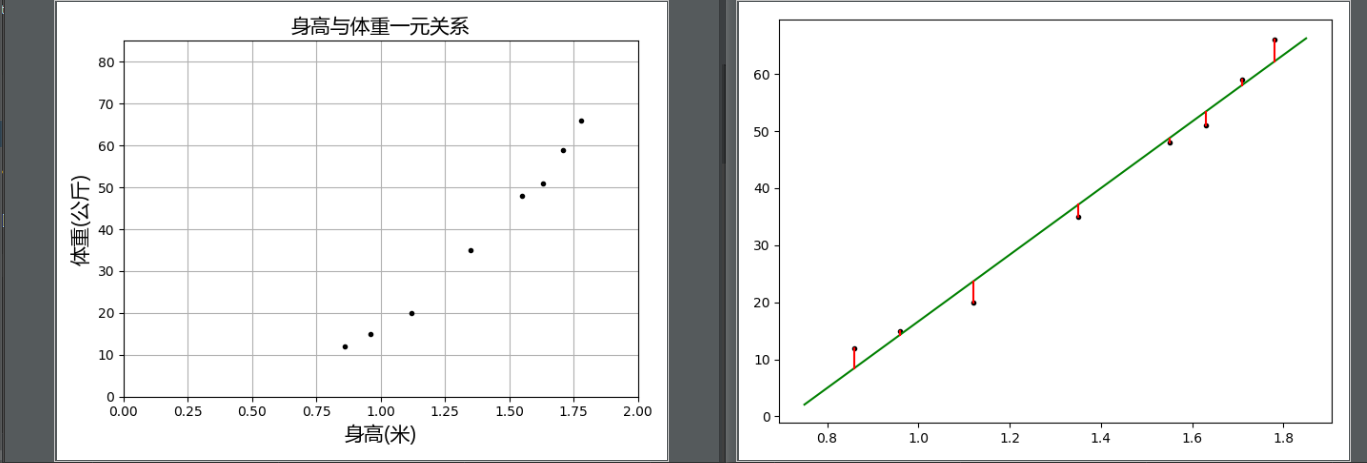身高、年龄与体重的二元回归
运行结果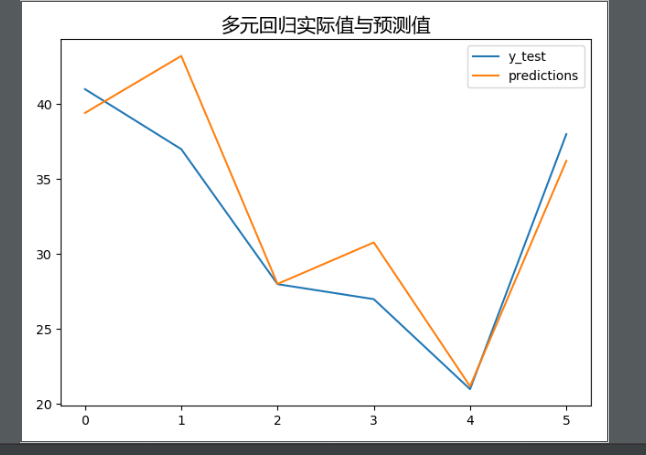身高与体重高次回归
运行结果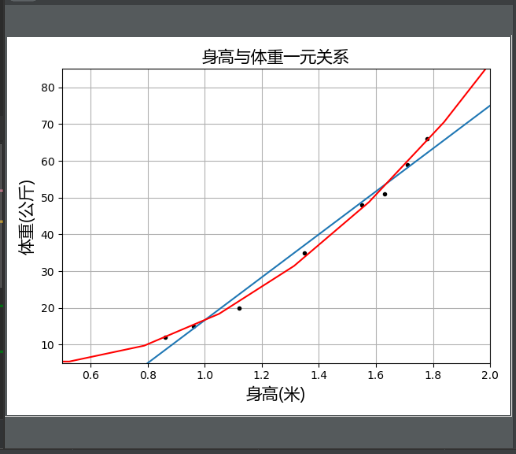房价预测
代码
  # -*-coding:utf-8-*-
import matplotlib.pyplot as plt
import pandas as pd
from sklearn.linear_model import LinearRegression
import numpy as np

# 输入文件，将房间大小和价格的数据转成scikitlearn中LinearRegression模型识别的数据
def get_data(file_name):
X_parameter = []
Y_parameter = []
for single_square_meter, single_price_value in zip(data['square_meter'], data['price']):
X_parameter.append([float(single_square_meter)])
Y_parameter.append([float(single_price_value)])
return X_parameter, Y_parameter

# 线性分析
def line_model_main(X_parameter, Y_parameter, predict_square_meter):
# 模型对象
regr = LinearRegression()
# 训练模型
regr.fit(X_parameter, Y_parameter)
# 预测数据
predict_outcome = regr.predict(predict_square_meter)
predictions = {}
# 截距值
predictions['intercept'] = regr.intercept_
# 斜率值
predictions['coefficient'] = regr.coef_
# 预测值
predictions['predict_value'] = predict_outcome
return predictions

# 显示图像
def show_linear_line(X_parameter, Y_parameter):
# 构造模型对象
regr = LinearRegression()
# 训练模型
regr.fit(X_parameter, Y_parameter)
# 绘制已知数据的散点图
plt.scatter(X_parameter, Y_parameter, color='blue')
# 绘制预测直线
plt.plot(X_parameter, regr.predict(X_parameter), color='red', linewidth=4)
plt.title('Predict the house price')
plt.xlabel('square meter')
plt.ylabel('price')
plt.show()

# 主函数
def main():
# 读取数据
X, Y = get_data('data/house_price.csv')
# 获取预测值，这里我们预测700平英尺的房子的房价
predict_square_meter = np.array([300, 400]).reshape(-1, 1)
result = line_model_main(X, Y, predict_square_meter)
for key, value in result.items():
print('{0}:{1}'.format(key, value))
# 绘图
show_linear_line(X, Y)

if __name__ == '__main__':
main()

  运行结果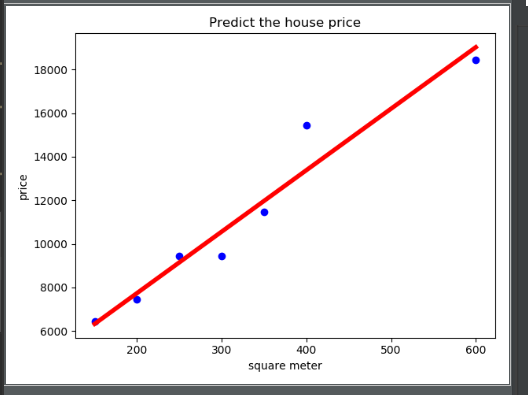产品销量与广告多元回归
代码
  # -*-coding:utf-8-*-
# 导入模块
import pandas as pd
import numpy as np
import matplotlib.pyplot as plt
from sklearn.linear_model import LinearRegression
from sklearn.model_selection import train_test_split

# 使用pandas读入数据

# 转换数据
X = data[feature_cols]
y = data['sales']

# 划分训练集和测试集
X_train, X_test, y_train, y_test = train_test_split(X, y, test_size=.4, random_state=0)

# 循环多元回归模型
linreg = LinearRegression()
model = linreg.fit(X_train, y_train)
print(model)
print(linreg.intercept_)
print(linreg.coef_)

# 预测
y_pred = linreg.predict(X_test)
print(y_pred)

# 使用图形来对比预测数据与实际数据之间的关系
plt.figure()
plt.plot(range(len(y_pred)), y_pred, 'b', label='predict')
plt.plot(range(len(y_pred)), y_test, 'r', label='test')
plt.legend(loc='upper right')
plt.xlabel('the number of sales')
plt.ylabel('value of sales')
plt.show()

# 模型验证
sum_mean = 0
for i in range(len(y_pred)):
sum_mean += (y_pred[i] - y_test.values[i]) ** 2
sum_erro = np.sqrt(sum_mean / 50)
print('RMSE by hand:', sum_erro)

  运行结果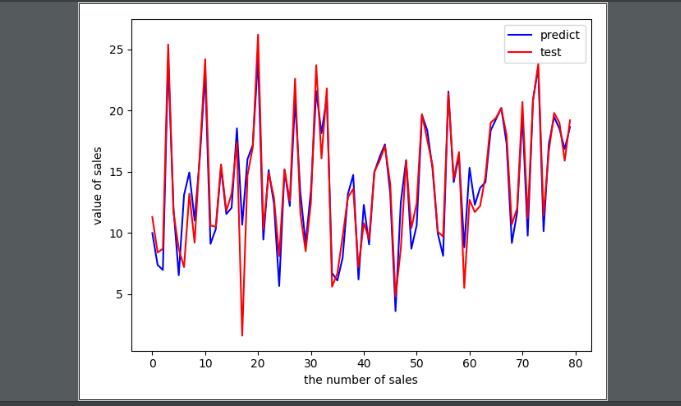补充说明
参考书为《Python3数据分析与机器学习实战》，对部分错误修改具体数据集和代码见我的Github，欢迎Star或者Fork 
展开全文一元回归
• PAGE 1 电脑输出的多元回归分析结果中 衡量拟合公式优劣的4类指标 ? 散点图及其一元回归拟合分析是常用的科研手段是寻找变量之间相互关系因果关系线索等的重要手段自然界变量之间的关系极其复杂两个变量之间可以是...
• 在大多数的实际问题中，影响因变量的因素不是一个而是多个，我们称这类回问 题为多元回归分析。
• 基于多重多元回归分析的单路口流量分析模型，亢旭东，许岷，在我国经济飞速发展之后，车辆的保有量和使用率也飞速提升，而城市交通堵塞极大地妨碍了城市居民的正常出行。本文利用多重多元线...